## A beam of light has a wavelength of 620 nm in vacuum. a. What is the speed of this light in a liquid whose index of refraction at this

Question

A beam of light has a wavelength of 620 nm in vacuum.
a. What is the speed of this light in a liquid whose index of refraction at this wavelength is 1.56? (Use 3.00×108 m/s for the speed of light in a vacuum.)
b. What is the wavelength of these waves in the liquid?

in progress 0
6 months 2021-07-30T08:10:19+00:00 1 Answers 12 views 0

1. Explanation:

It is given that,

Wavelength of a beam of light in vacuum,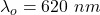(a) Let v is the speed of light in a liquid whose index of refraction at this wavelength is 1.56.

We know that,

Refractive index,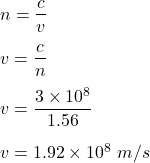(b) The wavelength of light in material,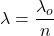Let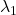is the wavelength of these waves in the liquid. So,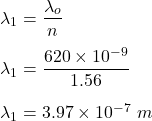Hence, this is the required solution.